# Horizons MathAlpha Omega Publication’s Horizons Math is a traditional math program for kindergarten through sixth grade. They also publish a Pre-Algebra course for junior high that I review elsewhere

In Horizons Math, the teacher handbook is the primary component of the program although each level does have two full-color student workbooks.

The teacher handbook outlines every step of each lesson, listing objectives, materials needed, stories, poems, and games. Some preparation time is needed, and lessons must be taught.

Horizons Math uses a variety of manipulatives throughout all levels, although far more in the early grades than in fifth and sixth grades. Among the manipulatives used are dominoes, counters, play money, place value materials, a flannel board with numbers, abacus, beads, and flashcards. You will also use household items such a calendar, an egg carton, a ruler, and straws. For the most part, these are things you can easily find or make yourself or they are relatively inexpensive items. Base Ten Blocks used at upper levels would be one of the more costly items. Charts at the front of each level’s teacher handbook list manipulatives to be used and lessons for which they are to be used. Charts also show which manipulatives are essential and which are optional.

Each lesson has instruction on a new concept plus practice or review of previously-learned concepts. This continual practice and review marks this as a “spiral” curriculum.

Every lesson includes a number of activities that require interaction between teacher and student, often with hands-on materials. For example, one lesson in the first-grade program has paper-and-pencil work with a "hundreds chart," a regrouping demonstration with place value manipulatives, oral number-chart work, time-telling practice using small clocks, written place value practice, addition practice, writing the words for large numbers, and word problems.

Alpha Omega Publications (AOP) explains their scope and sequence as well as their course layout in great detail at the beginning of each teacher handbook. The readiness evaluation that is also found there will help ensure that you are selecting the correct level in the program. Readiness evaluations are also available for free at www.aophomeschooling.com/diagnostic-tests.

The teacher handbook is very well designed with each part of the lesson clearly labeled. Activity instructions are numbered and spaced so they are easy to locate and read quickly. All instruction is provided through one-on-one teacher instruction, demonstrations, and hands-on activities.

Students have two separate workbooks (each about one-half inch thick) to cover each level. This is a lot of workbook pages, especially for kindergarten—generally, two to three per lesson depending upon grade level. But the workbooks are appealingly designed with full color, large print, and variety in the layout. Illustrations, puzzles, and lesson explanations take up some space as well, so the overall impact is not overwhelming.

I suspect that many parents will be tempted to hand their children the workbooks and ignore the teacher handbooks, but there are important teaching instructions in the handbooks you should not skip. You should review the lesson plans and determine how much of each presentation is useful for each student.

Supplemental, reproducible worksheets are also included in the teacher handbook, clearly indicating lessons to which they correlate. You can purchase the worksheets as a separate packet if you prefer not to photocopy pages from the teacher handbook. Periodic tests are in student workbooks, and answer keys to workbook pages, including tests, are in the teacher handbook.

Each level goes beyond most other programs, spending more time on the development and practice of concepts and skills. The scope and sequence is purposely advanced. The Horizons Math program was not written to align with the Common Core Standards. However, it seems to cover the standards although sometimes at a grade level earlier than required by the standards themselves.

AOP’s educational philosophy is also very evident in this program. They believe repetition and review are essential until a subject has been mastered to the point where it becomes second nature. They view math as both a basic functional skill and a communications skill that develops precision in thinking. Within this framework that emphasizes mental discipline, they have done an excellent job of breaking tasks down into manageable increments while also building in methods that address the needs of various learning styles.

However, this also means that you might not need to use everything in each lesson with each of your children. Some children won't need all of the multi-sensory instruction and others don't need all of the practice and review (in spite of instructions to the contrary in the teacher handbooks). Exercise your own discretion as to what to use and what to skip.

Quarterly tests and a final in grades four through six, plus answer keys for workbooks, worksheets, and tests are all in the teacher handbook. Except for Horizons Math K, there is also a test after every ten lessons in the student workbooks.

This program was designed very much with home educators in mind, so there are very few classroom-only type activities that must be adapted or skipped.

### Horizons Math K

The Horizons Math K program follows an advanced scope and sequence, closer to some publishers’ first-grade programs. Students perform addition and subtraction (two digits plus or minus one digit) with no regrouping by the end of the year. However, lessons are taught with visual aids and manipulatives to better help young children grasp concepts. Time, money, measurement, ordinal numbers, and introductory fractions are among other concepts covered. For home educators who want an advanced academic math program for kindergarten, this is a practical solution.

### Horizons Math 1

The first-grade program begins with concepts such as place value and counting by twos and fives—all within the first ten lessons. Addition advances through addition of triple-digit numbers with carrying from the ones column. Subtraction works up through three-digit numbers but without borrowing. In addition to basic number concepts (e.g., counting, addition, subtraction, place value), this level teaches time, money, calendar use, measurement, fractions, sets, shapes, bar graphs, and estimation.

A solid foundation in number recognition and number values along with other concepts such as colors and shapes is essential before beginning this level. (This foundation is laid in Math K.) If children are weak in some areas, extra lessons in the handbook can be used to cover some topics. However, some children in first grade might need to start with Math K. Choose levels according to appropriate skill levels rather than equating them to grade levels.

### Horizons Math 2

Math 2 expects that children have learned two-digit addition and subtraction with carrying, but it still reviews the concepts then moves on to larger numbers. Multiplication facts for 1 through 10 are taught along with place value, sets, correspondence, cardinal and ordinal numbers, shapes, graphs, fractions (½, ⅓, ¼), measurement, temperature, estimation, ratio, the calendar, time, money, area, perimeter, volume, and decimals (in money).

### Horizons Math 3

Math 3 covers the same topics as Math 2 but at more challenging levels; e.g. multiplication teaches up through four-digit multipliers and division works up through two-digit divisors with remainders. Algebraic thinking is introduced with equations like n + 5 = (7 + 2) + 4.

### Horizons Math 4

The readiness test at the front of the book will help you know whether or not your child is able to work at this level. It asks students to reduce fractions, multiply four-digit numbers by multiples of ten, perform short division, compare values of fractions with unlike denominators, round off numbers, understand ratio, add fractions with common denominators, and solve simple, algebraically-expressed addition equations.

Among concepts covered by the end of the course are long division with two-digit divisors, adding and subtracting fractions with unlike denominators, converting fractions to decimals, adding and subtracting decimals, metric measurement, and multiplying or dividing to find equal ratios. Time, money, geometry, and graphs are also covered.

Lessons are designed to be presented by the teacher as with lower levels, but students should be able to do much of their work independently. Materials or supplies needed are listed, and you might have to plan ahead to procure some of these. One lesson describes a bingo game for the teacher to construct, but other than that, most materials are much more standard—counters, flash cards, rulers, Base Ten Blocks, a clock, and play money. While much of the lesson activity takes place within the two student workbooks for this level, there are additional activities such as mental math or manipulative work described in the lesson plans. About every other lesson uses a worksheet for which reproducible masters are found in the teacher handbook.

### Horizons Math 5

Among concepts on the readiness test for Math 5 (which students are expected to know before beginning this course) are division of two-digit divisors into dollar amounts with decimals; acute angles; diameters and radii of circles; similar and congruent figures; simple perimeter, area, and volume; ratios; addition of fractions with unlike denominators, addition and subtraction of mixed numbers, decimal values, and metric measurements. As with earlier levels, there is a great deal of review, so if your child has not yet covered all of these concepts, he or she might be able to pick them up through the review that is built into Math 5.

This course continues to stress both computation skills and understanding of concepts. Among concepts taught by the end of this course are multiplying three-digit by three-digit numbers, values of numbers with exponents, finding averages, division by two-digit divisors, least common multiples, multiplying and dividing fractions, all four functions applied to decimal numbers, percents, and probability. Calculators are used, primarily for checking answers.

### Horizons Math 6

Students beginning this level are expected to know how to work with fractions, decimals, and percents. They should also have been introduced to concepts such as congruency, similarity, diameter and chords of circles, and different types of averages. However, the continual review and spiral approach used throughout the program mean that these concepts are reviewed or retaught at this level. Still, the program moves beyond the level of most other sixth grade courses. For example, Saxon Math 7/6 introduces the idea of ratio while Horizons Math 6 goes further, teaching cross multiplication to solve for n. Geometry coverage is more complex with students learning to construct geometric figures using a compass and straightedge. Students continue to work with fractions, decimals, and percents. Consumer math topics such as check writing, banking, budgeting, and computing interest are covered along with more advanced equations, graphs, measurement, and problem solving.

Students who complete this course should be prepared for Horizons Pre-Algebra.

You might want to check out the premade lesson plans from Homeschool Planet that are available for Horizons Math.Find lesson plans available for this product at Homeschool Planet. Sign up for a 30-day FREE trial.

### Pricing Information

When comparison prices appear, please keep in mind that they are subject to change. Click on links where available to verify price accuracy.

#### Horizons Mathematics Kindergarten Complete Set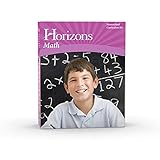#### Horizons Grade 6 Manipulative Kit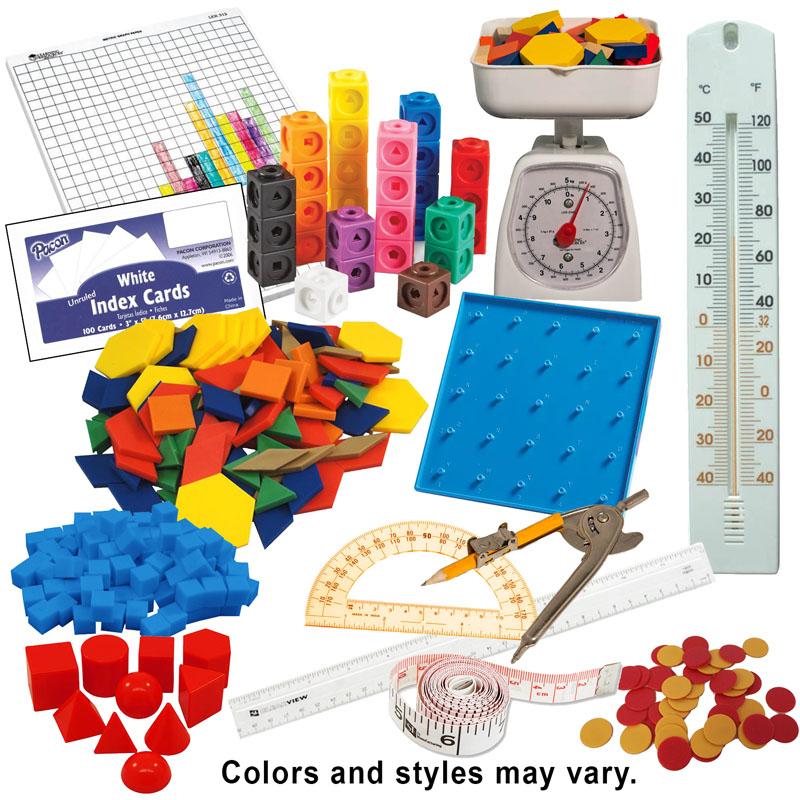#### Horizons Grade 1 Manipulative Kit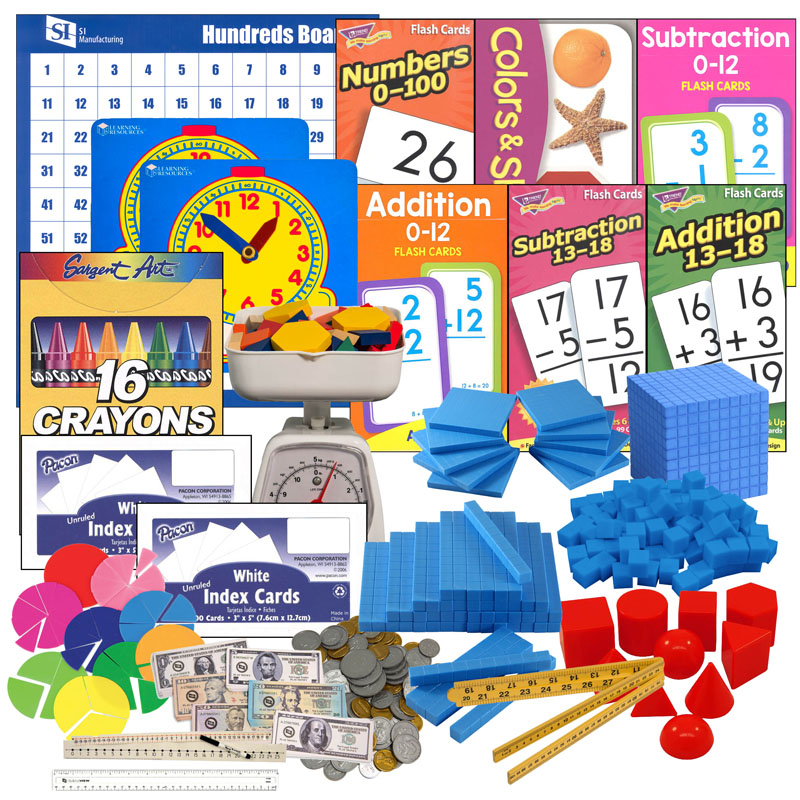#### Horizons Mathematics Grade 3: Home School Curriculum Kit (Lifepac)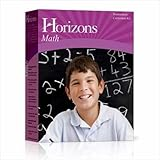#### Horizons Mathematics Grade 4 Boxed Set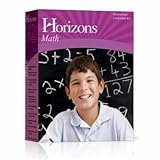#### Horizons Mathematics Grade 1 Set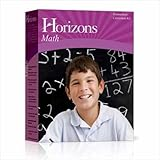#### Horizons Grade 5 Manipulative Kit+Wooden P/B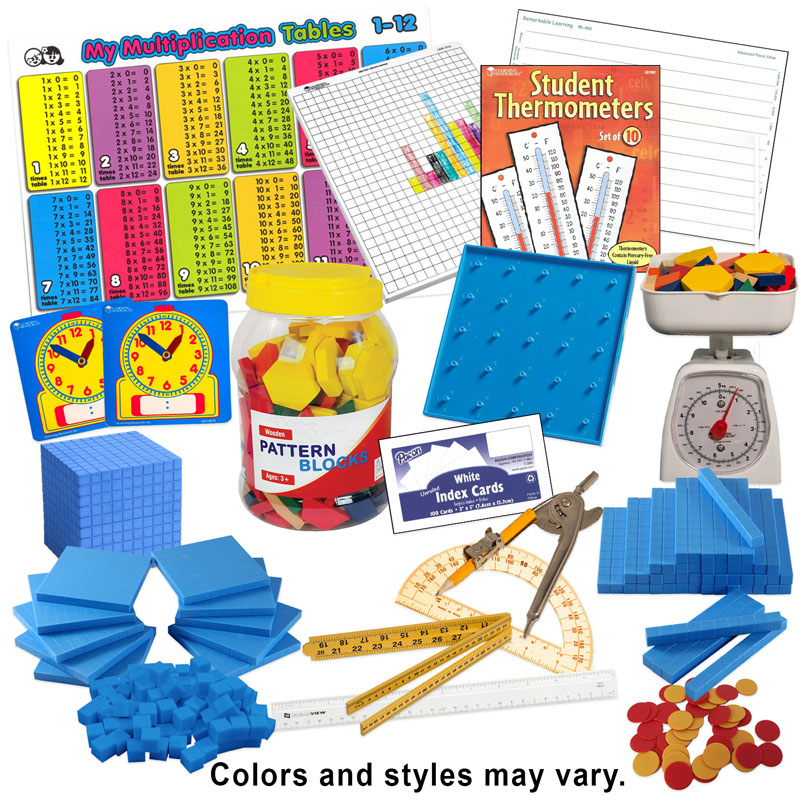#### Horizons Grade 3 Manipulative Kit#### Horizons Grade 2 Manipulative Kit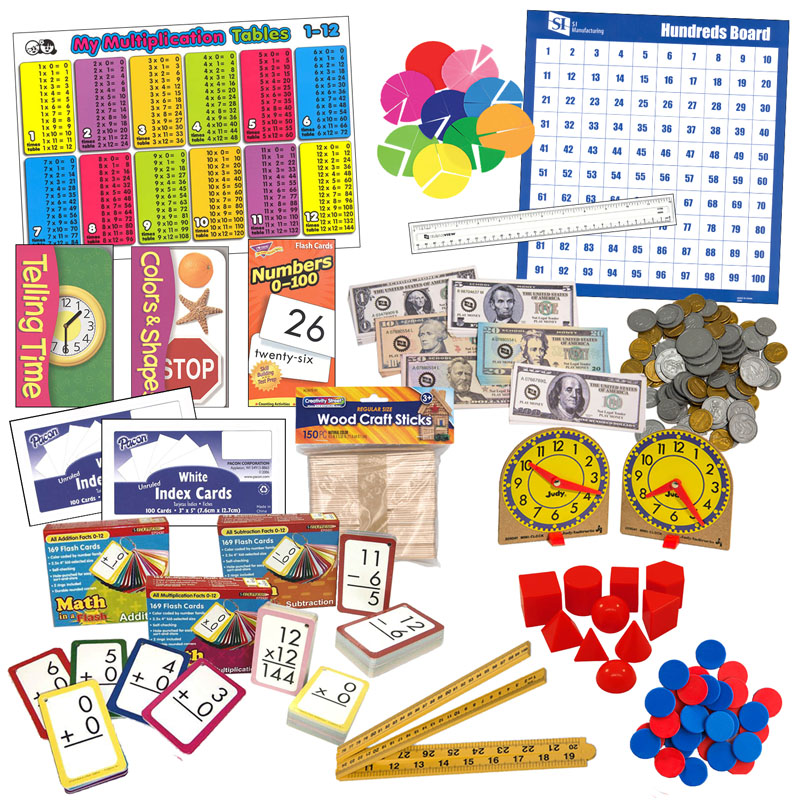#### Horizons Grade K Manipulative Kit#### Horizons Math 2 Boxed Set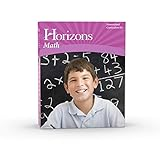#### Horizons Math 6 Boxed Set#### Horizons Math 5 Boxed SetGet a FREE subscription to Cathy's E-Newsletter

### My Lists

Save reviews to lists to guide curriculum choices. Register or log in to start your first list.

### Instant Key

• Need For Parent or Teacher Instruction: moderate to high
• Learning Environment: group or one-on-one
• Educational Methods: traditional activity pages or exercises, interactive, hands-on